Select Page

# KINETIC FRICTION:

The Complete GuideIf you want in-depth knowledge on kinetic friction, then you NEED this guide.

Why?

Well, It covers everything you need to know about kinetic friction in one single article.

With simplified definitions and explanations, it is packed with illustrative diagrams to enhance understanding.

Bottom line:

If you want to get the most out of kinetic friction, you’ll love this guide.### CHAPTER 1

KINETIC FRICTION: Definition and Explanation### CHAPTER 2

Examples of Kinetic Friction### CHAPTER 3

Factors affecting Kinetic Friction### CHAPTER 4

Calculations on Kinetic Friction

## Contents### CHAPTER 1

KINETIC FRICTION: Definition and Explanation### CHAPTER 2

Examples of Kinetic Friction### CHAPTER 3

Factors affecting Kinetic Friction### CHAPTER 4

Calculations on Kinetic Friction

CHAPTER 1

KINETIC FRICTION: Definition and Explanation# What is kinetic friction?

Kinetic friction is the friction between two surfaces that moves or slides relative to each other.

It is the friction that exists between the contact surface of moving objects.

Kinetic friction is also called sliding friction or dynamic friction.

When a body at rest is set in motion, the friction force in action changes from static friction to kinetic friction.

Let us buttress this point with two case studies.

CASE 1

Consider a lady riding a bicycle in the forward direction.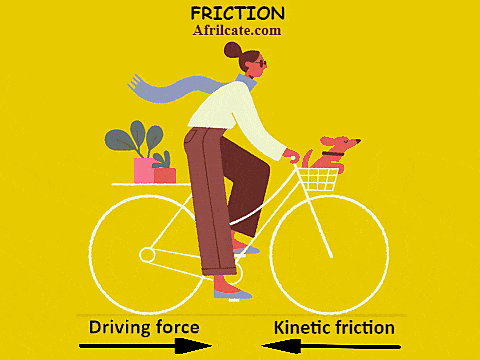Kinetic friction exists between the tires of the bicycle and the surface upon which she rides.

This friction opposes the forward motion and tries to bring the bicycle to a complete stop.

To maintain this motion, the driving force of the bicycle must be greater than the kinetic friction.

The more force exerted on the bicycle pedals by her feet, the greater the driving force.

You should know that friction is a contact force and it always act in the opposite direction to motion.

By contact force, it means that friction only exists between surfaces that are physically in contact.

CASE 2

An object at rest weighing 200N is to be pushed across a surface with friction resistance of 100N.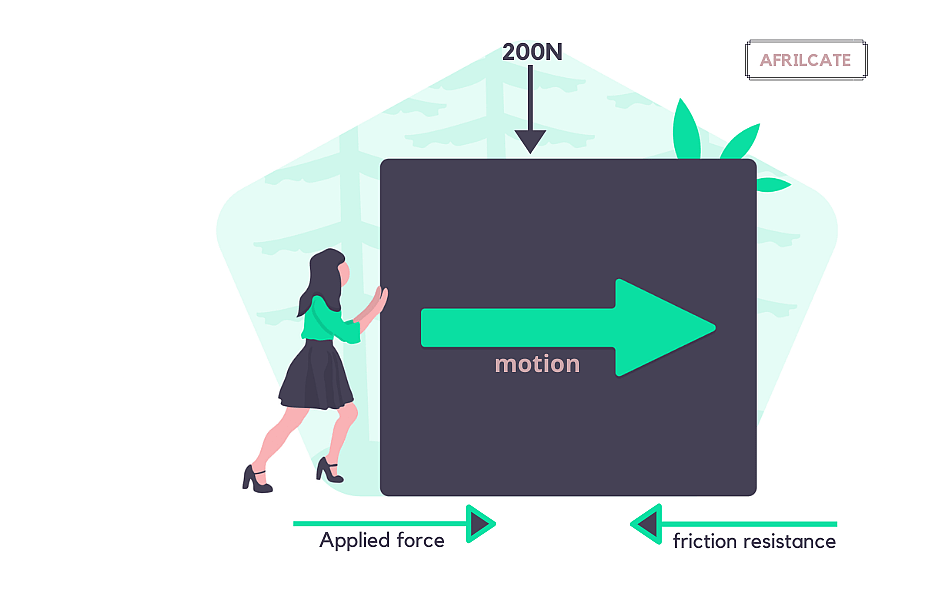Similar with the previous case, the applied force must be greater than the friction resistance before motion can be initiated. This means that the push exerted must be greater than 100 N.

The friction force acting on the object when at rest is the static frictional force.

The static frictional force is always greater than the kinetic frictional force because a stronger adhesive bond is formed between stationary surface compared to surfaces in relative motion.

Let’s take a quick look at the friction plot.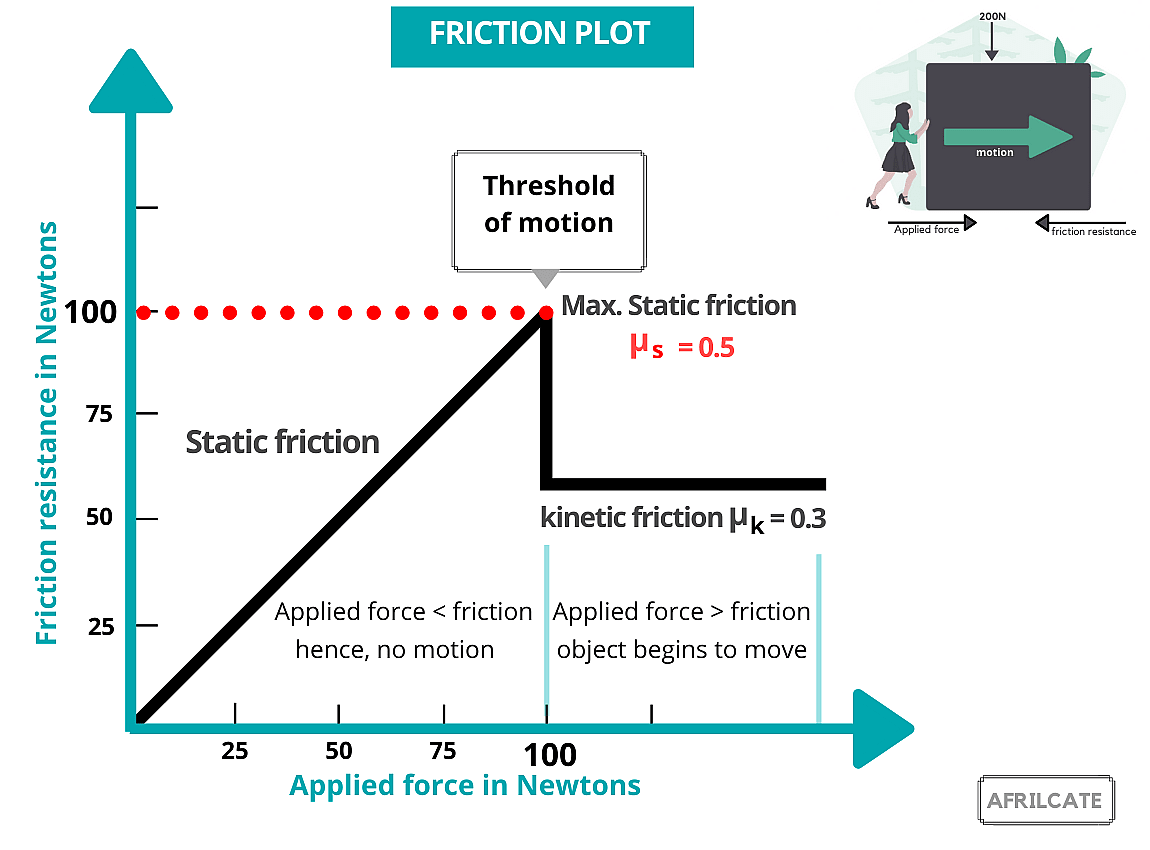From the plot, you will observe that the object begins to move when the applied force exceeds the maximum static threshold.

The sharp downward drop from the plot shows a reduction in friction force once the applied force exceeds the threshold of motion.

Generally, the terms “kinetic”, “sliding” and “dynamic” all refer to objects in motion.

For friction, they all mean the same thing and are used interchangeably.

That’s why some text calls it sliding friction, others use dynamic friction.

But for this guide, let’s stick with the word kinetic friction.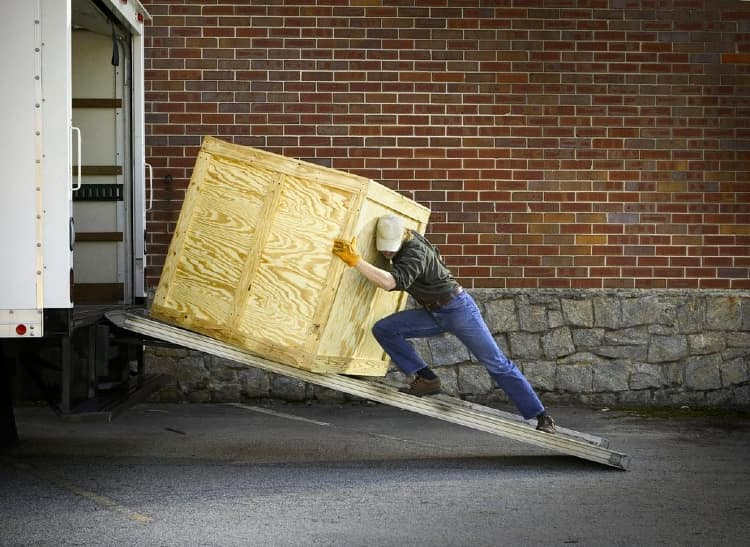Kinetic friction is slightly different from rolling friction.

While kinetic friction exists between surfaces that move or slides relative to each other, rolling friction resists the motion of a rolling body on a surface.

CHAPTER 2

Examples of Kinetic Friction# Examples of kinetic friction?

There are various examples of Kinetic friction in everyday life.

Out listed below are some common examples:

1. A book being slid across a table.
2. An ice skater skating on ice.
3. A car moving on a road.
4. A pressing iron being pushed across material.
5. Movement of door hinges when the door is opened or closed.CHAPTER 3

Factors affecting Kinetic Friction# Factors affecting kinetic friction

There are three factors that affects kinetic friction. They include:

1. Nature of surfaces in contact
2. Weight of object
3. Coefficient of Kinetic friction

Apparently, the factors affecting kinetic friction is the same as that affecting friction generally.

The only difference is the specific reference given to the friction coefficient.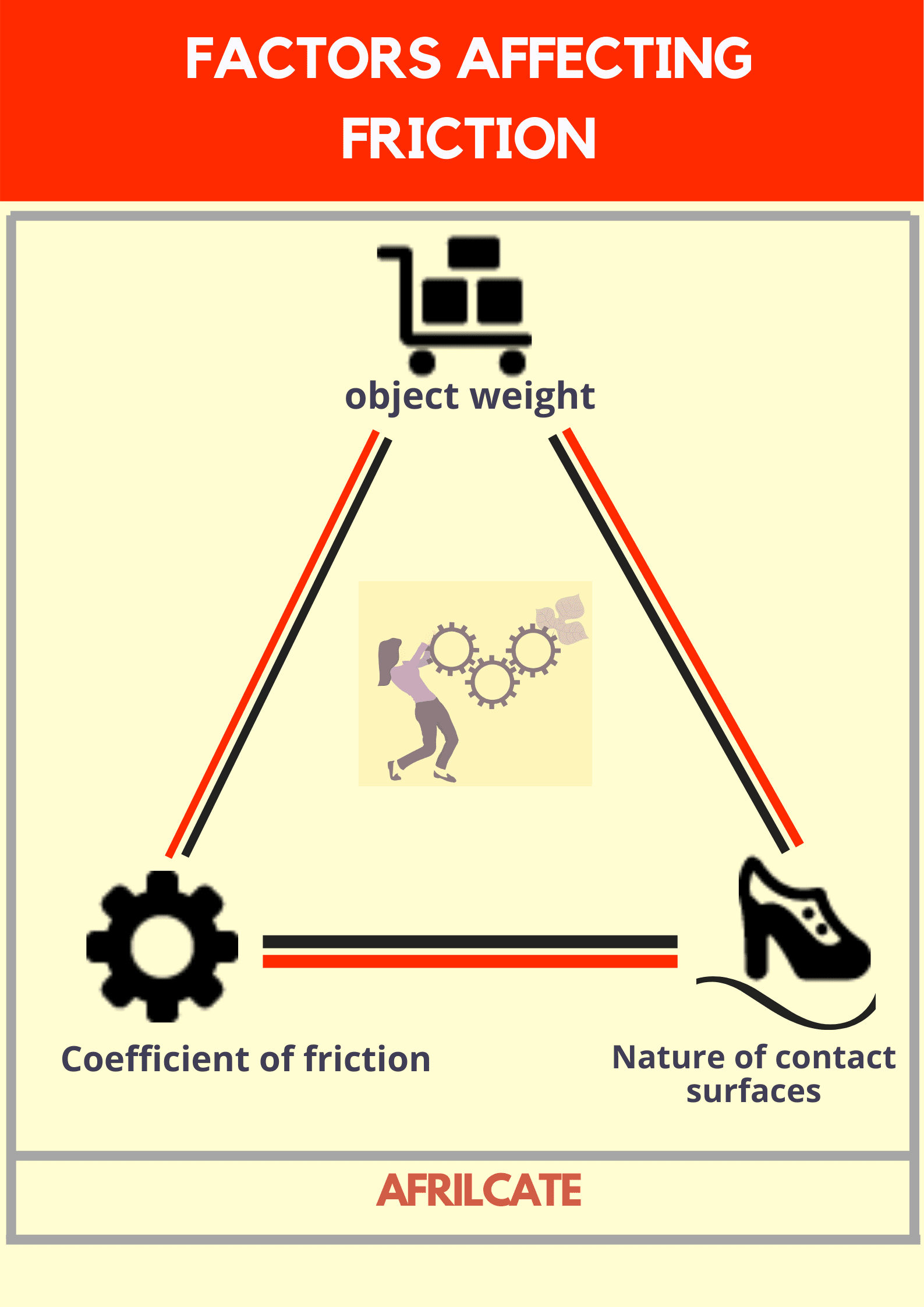Let’s see how these three factors affect kinetic friction.

## Nature of surfaces in contact

To a large extent, kinetic friction depends on the nature of the surfaces in contact.

No matter how smooth a surface might appear, it still contains traces of minute rough planes or unevenness.

This unevenness might not be visible to the human eyes due to its tiny proportion but can be detected with a microscopic lens.Microscopic view of two surfaces in contact

These tiny rough planes are called surface irregularities.

The interlocking of surface irregularities hinders the relative motion of objects and gives rise to kinetic friction.

Generally, the roughness or smoothness of the contact surface affects kinetic friction.

They are larger number of irregularities on rough surfaces.

If two surfaces in contact are extremely rough, the surface irregularities will easily be locked together.

This will strengthen the intermolecular bond due to adhesion and further prevent the sliding action of the object.

The interlocking of surface irregularities and adhesive bond is strongest in static friction and weakest in rolling friction.

Smooth surfaces offer a lesser resistance to kinetic friction.

For practical purposes, try pushing a box along a tiled surface, then repeat the same action on a tarred road.

Which of these two surfaces do you think will require more push?

Since the tarred road rougher than the tiled floor, you will exert a greater force to cover the same distance with the tiled floor.

Therefore, the friction force is greater if the surface is rough.

Sticky surfaces also hinder motion and this enhances kinetic friction.

That’s why we expel more energy when walking on a muddy floor.

## Weight of object

The normal force acting on an object depends on the weight of the object.

Normal forces are support forces that assist an object to stay at the surface of another object.

When a body is moving over a horizontal surface, it presses down against the surface with a force equal to its weight, the pull of gravity.An increase in the weight of an object will cause an increase in the resistance offered to motion.

And a decrease in weight will offer lesser resistance and reduced kinetic friction.

Forcefully moving heavy objects over  rough surfaces can lead to the dislodging of the material particles, a process called surface abrasion.

## Coefficient of Kinetic Friction

The coefficient of kinetic friction, denoted as µk is a system property.

Coefficient of friction is a numerical value that quantifies the intensity with which two sliding or contact surfaces grip each other.

The kinetic friction coefficient is a measure of the material grip between a moving object and its contact surface.

Since static friction force is greater than kinetic friction force, static friction coefficient has a greater value than kinetic friction coefficient.

Rolling friction coefficient possesses the least value due to the small contact area between the rolling object and the surface.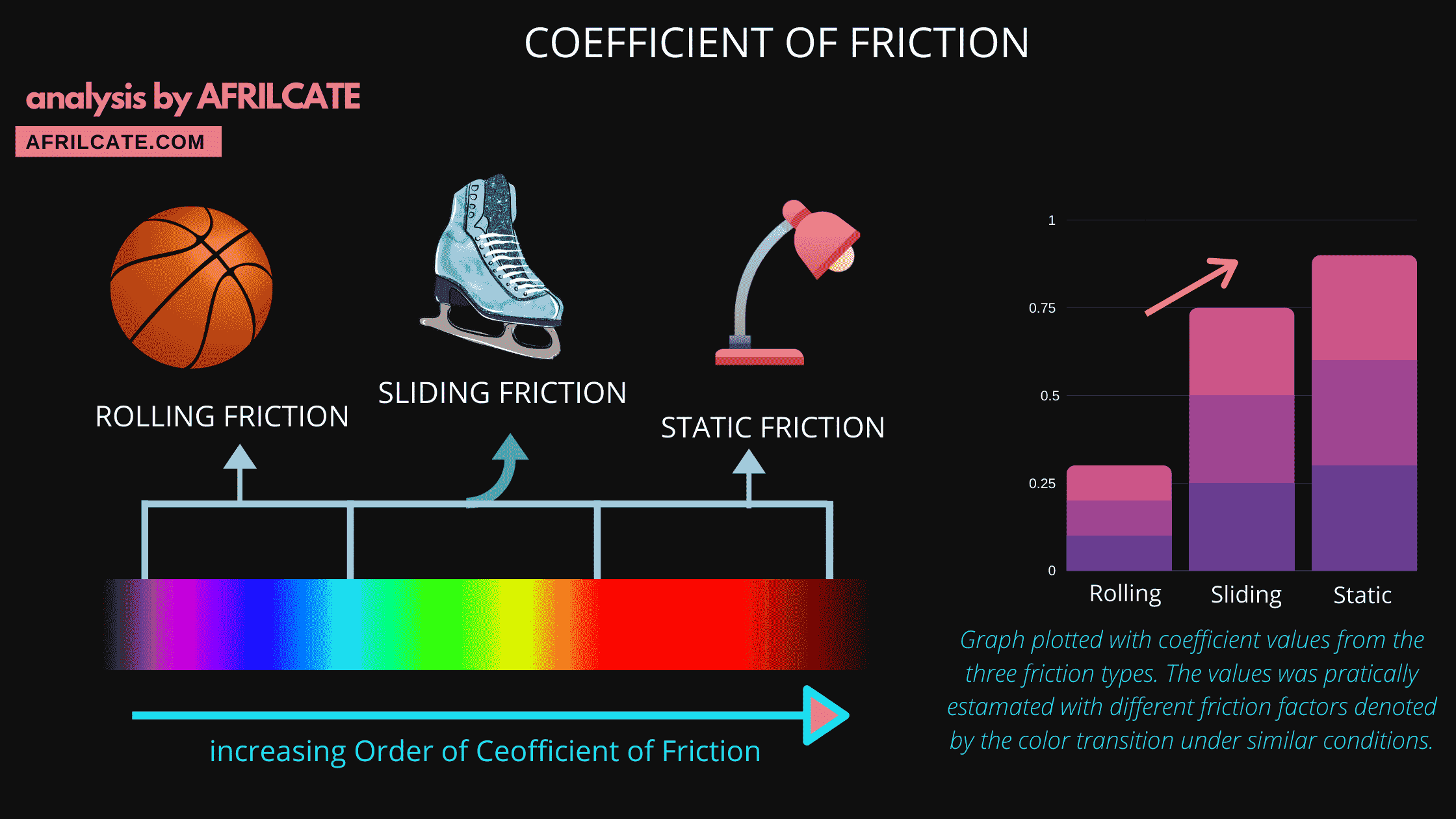Graph plotted with coefficient values from the three friction types.

The values were practically estimated under similar conditions. These conditions are denoted by the color transition in each column of the friction coefficient plot.

Friction Coefficient Comparison

Stanley Udegbunam (Afrilcate)

The higher the coefficient of friction, the higher the frictional force.

Similarly,

The higher the kinetic coefficient of friction, the higher the kinetic friction force.

CHAPTER 4

Calculations on Kinetic Friction# Calculations on kinetic friction

The formula for kinetic friction is given as the product of the coefficient of kinetic friction and normal force.

 Fk = µk N

Where,

• Fk is the force of kinetic friction
• µk is the coefficient of kinetic friction
• N is the normal force, sometimes it’s denoted by η

Normal force has the same magnitude as the weight.

It’s represented by the equation; N = m x g

If the object or plane is inclined at an angle (∅), the normal force becomes:

N = m gcos∅

Where m = mass of the object.

And g = acceleration due to gravity.

F and N are measured in units of force (such as Newton or pound).

Since friction is a force, the unit of the frictional force is the newton (N).

The coefficient of kinetic friction is unitless.

Example

A 3kg  stainles cup is pushed accross a horizontal glass table with µk = 0.6

Calculate the kinetic friction force given that g = 9.81 m/s2.

Solution

Fk = µkN

but N = m x g

Fk = µk mg

Fk = 0.6 x 3 x 9.81

Fk = 17.66 N

A total force of 20N is required to move a 5 kg block along a horizontal wooden surface.

If the acceleration due to gravity is estimated to be 10 m/s2, calculate the coefficient of kinetic friction.

X wrong!!

✔ Correct

X wrong!!

##### d.) none of the above

X wrong!!

want to know more about kinetic friction coefficient?

See our article: Coefficient of Kinetic Friction (Fully Explained)

##### DO YOU KNOW?

Just like motion, there are basic laws that governs friction.

These laws were postulated by Guillaume Amontons, a French Physicists who was a major pioneer in studying the problem of friction.

These laws are known as laws of friction.

### Now, Over to You…

There you have it: our guide on Kinetic Friction.

I hope you enjoyed it.

Now I’d like to hear what you have to say.

Are you know you conversant with outer friction types?

While reading this guide, were you in a static or kinetic position? ….or you transitioned across both positions?

Either way, Let me know by leaving a comment below.